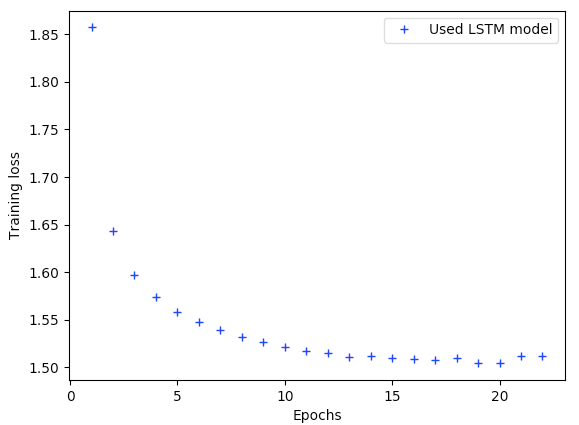# Introduction to Language Modelling and Deep Neural Network Based Text Generation

Source: Deep Learning on MediumIntroduction to Language Modelling and Deep Neural Network Based Text Generation

Introduction

NLP studies involve a number of important tasks like text classifications, sentiment analysis, machine translation, text summarization etc. One other core tasks of NLP is related with language modelling which involves generating text, conditioned on some input information. Before the recent advancement in deep neural network models, the most commonly used methods for text generation were either based on template or rule-based systems, or probabilistic language models such as n-gram or log-linear models [Chen and Goodman, 1996, Koehn et al., 2003]. Language Model is the task of predicting what word comes next or more generally, a system that assigns probability to a piece of a text sequence.N-gram is the simplest language model and its performance is limited by its lack of complexity. Simplistic models like this one cannot achieve fluency, enough language variation and correct writing style for long texts. For these reasons, neural networks (NN) are explored as the new main standard despite their complexity. And Recurrent Neural Networks (RNN) became a fundamental architecture for sequences of any kind. RNN is nowadays considered as the default architecture for text but RNNs have problems of their own: it cannot remember for long the content of the past and it struggles to create long relevant text sequences because of exploding or vanishing gradient problems. For these reasons, other architectures such as Long Short Term Memory (LSTM) [Alex Graves et all, 2014] and Gated Recurrent Units (GRU) [Kyunghyun Cho et al, 2014] were developed and became the state of the art solution for many language generation tasks. In this post, we will be using LSTM to generate sequences of text.

Language Model

Models that assign probabilities to sequences of words are called language models. There are primarily two types of Language Models:

1) Statistical Language Models: These models use traditional statistical techniques like N-grams, Hidden Markov Models (HMM) and certain linguistic rules to learn the probability distribution of words.

2) Neural Language Models: They use different kinds of Neural Networks to model language and have surpassed the statistical language models in their effectiveness.

N-Gram Models

We have described language models as calculating the probability of next word given a sequence of words. Let’s begin with the task of computing P(w|h), the probability of a word w given some history h. Suppose the history h is “its water is so transparent that” and we want to know the probability that the next word is “the”:

P(the|its water is so transparent that).

Instead of computing the probability of a word given its entire history, we can approximate the history by just the last few words. Below is the mathematical representation of different n-gram models: